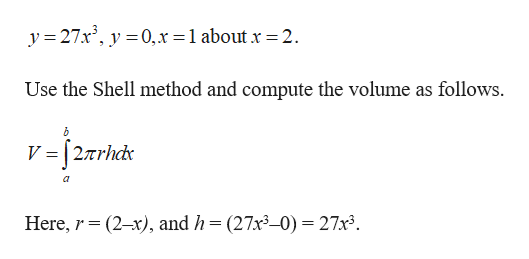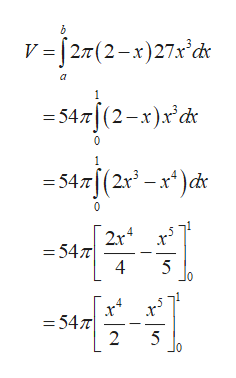# Find the volume V of the solid obtained by rotating the region bounded by the given curves about the specified line.y = 27x3,    y = 0,    x = 1;    about x = 2

Question
70 views

Find the volume V of the solid obtained by rotating the region bounded by the given curves about the specified line.

y = 27x3,    y = 0,    x = 1;    about x = 2
check_circle

Step 1

The given curves are,help_outlineImage Transcriptionclosey = 27x', y =0,x =1 about x = 2. Use the Shell method and compute the volume as follows. V = [2arhdx Here, r= (2-x), and h=(27x³_0) = 27x³. fullscreen
Step 2

That impli...help_outlineImage Transcriptioncloseма- V-[2т (2-х)27x d* 1. -54т[(2—к)x & = 54.7[(2r° - x*)dr 2r x — 54л 5 x* — 54т 2 х? 5 fullscreen

### Want to see the full answer?

See Solution

#### Want to see this answer and more?

Solutions are written by subject experts who are available 24/7. Questions are typically answered within 1 hour.*

See Solution
*Response times may vary by subject and question.
Tagged in

### Other